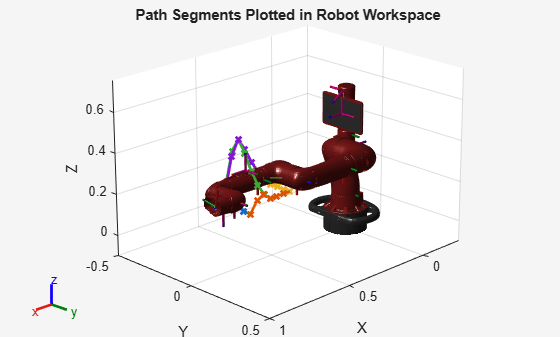# Manipulator Shape Tracing in MATLAB and Simulink

This example shows how to trace a predefined 3-D shape in space. Following a smooth, distinct path is useful in many robotics applications such as welding, manufacturing, or inspection. A 3-D trajectory is solved in the task space for tracing the MATLAB® membrane and is executed using the Sawyer robot from Rethink Robotics®. The goal is to generate a smooth path for the end effector fo the robot to follow based.

The Manipulator Shape Tracing in MATLAB and Simulink example shows how to generate a closely discretized set of segments that can then be passed to an inverse kinematics solver to be solved using an iterative solution. However, this example offers an an alternate approach to reduce computational complexity. This example splits paths segments into just a few discrete points and uses smoothing functions to interpolate between the waypoints. This approach should generate a smoother trajectory and improve run-time efficiency.

This example uses the Sawyer robot from Rethink Robotics®. Import the URDF file that specifies the rigid body dynamics. Set the `DataFormat` to use column vectors to define robot configurations. Simulink® uses column vectors. The task space limits are defined based on empirical data.

```sawyer = importrobot('sawyer.urdf'); sawyer.DataFormat = 'column'; taskSpaceLimits = [0.25 0.5; -0.125 0.125; -0.15 0.1]; numJoints = 8; % Number of joints in robot```

### Generate a Set of Task-Space Waypoints

For this example, the goal is to get a set of path segments that trace the MATLAB® membrane logo. The membrane surface and the path segments are generated as cell arrays using the helper function `generateMembranePaths`. To visualize the paths overlaid on the surface, plot the surface using `surf` and the path segments by iterating through the path segment cell array. You can increase `numSamples` to sample more finely across the surace.

```numSamples = 7; [pathSegments, surface] = generateMembranePaths(numSamples, taskSpaceLimits); % Visualize the output figure surf(surface{:},'FaceAlpha',0.3,'EdgeColor','none'); hold all for i=1:numel(pathSegments) segment = pathSegments{i}; plot3(segment(:,1),segment(:,2),segment(:,3),'x-','LineWidth', 2); end hold off```To ensure that the robot can trace the output, visualize the shape in the robot workspace. Show the `sawyer` robot and plot the line segments in the same figure.

```figure show(sawyer); hold all for i=1:numel(pathSegments) segment = pathSegments{i}; plot3(segment(:,1),segment(:,2),segment(:,3),'x-','LineWidth',2); end view(135,20) axis([-1 1 -.5 .5 -1 .75]) hold off```### Create an Inverse Kinematics Solver

Create an inverse kinematics (IK) using the loaded `sawyer` rigid body tree . It is initially configured with a uniform set of weights, using the home configuration as the initial guess. Set the initial guess to the home configuration and the pose tolerances with uniform weights. The end effector for IK solver is the `'right_hand'` body of the robot.

```ik = inverseKinematics('RigidBodyTree', sawyer); initialGuess = sawyer.homeConfiguration; weights = [1 1 1 1 1 1]; eeName = 'right_hand';```

### Convert Task-Space Waypoints to Joint-Space Using Inverse Kinematics

Use the inverse kinematics solver to generate a set of joint space waypoints, which give the joint configurations for the robot at each point of the generated `pathSegments`. Each joint-space segment is filed into a matrix, `jointPathSegmentMatrix`, which is passed to the Simulink model as an input.

```% Initialize the output matrix jointPathSegmentMatrix = zeros(length(pathSegments),numJoints,numSamples); % Define the orientation so that the end effector is oriented down sawyerOrientation = axang2rotm([0 1 0 pi]); % Compute IK at each waypoint along each segment for i = 1:length(pathSegments) currentTaskSpaceSegment = pathSegments{i}; currentJointSegment = zeros(numJoints, length(currentTaskSpaceSegment)); for j = 1:length(currentTaskSpaceSegment) pose = [sawyerOrientation currentTaskSpaceSegment(j,:)'; 0 0 0 1]; currentJointSegment(:,j) = ik(eeName,pose,weights,initialGuess); initialGuess = currentJointSegment(:,j); end jointPathSegmentMatrix(i, :, :) = (currentJointSegment); end```

Use the `shapeTracingSawyer` model to execute the trajectories and simulate them on a kinematic model of the robot.

`open_system("shapeTracingSawyer.slx")`

The Simulink model has two main parts:

1. The Trajectory Generation section takes the matrix of joint-space path segments, jointPathSegmentMatrix, and converts the segments to a set of discretized joint-space waypoints (joint configurations) at each time step in the simulation using a MATLAB function block. The Polynomial Trajectory Block converts the set of joint configurations to a smoothed joint space B-spline trajectory in time.

2. The Robot Kinematics Simulation section accepts the joint-space waypoints from the smoothed trajectory and computes the resulting end-effector position for the robot.

#### Trajectory Generation#### Robot Kinematics Simulation### Execute Joint-Space Trajectories in Simulink

Simulate the model to execute the generate trajectories.

`sim("shapeTracingSawyer.slx")`

### View Trajectory Generation Results

The model outputs the robot joint configurations and the end-effector positions along each smoothed path trajectory. To work easily with MATLAB plotting tools, reshape the data.

```% End effector positions xPositionsEE = reshape(eePosData.Data(1,:,:),1,size(eePosData.Data,3)); yPositionsEE = reshape(eePosData.Data(2,:,:),1,size(eePosData.Data,3)); zPositionsEE = reshape(eePosData.Data(3,:,:),1,size(eePosData.Data,3)); % Extract joint-space results jointConfigurationData = reshape(jointPosData.Data, numJoints, size(eePosData.Data,3)); ```

Plot the new end-effector positions on the original membrane surface.

```figure surf(surface{:},'FaceAlpha',0.3,'EdgeColor','none'); hold all plot3(xPositionsEE,yPositionsEE,zPositionsEE) grid on hold off```### Visualize Robot Motion

In addition to the visualization above, the tracing behavior can be recreated using the Sawyer robot model. Iterate through the joint configurations in `jointConfigurationData` to visualize the robot using `show` and continuously plot the end-effector position in 3-D space.

```% For faster visualization, only display every few steps vizStep = 5; % Initialize a new figure window figure set(gcf,'Visible','on'); % Iterate through all joint configurations and end-effectort positions for i = 1:vizStep:length(xPositionsEE) show(sawyer, jointConfigurationData(:,i),'Frames','off','PreservePlot',false); hold on plot3(xPositionsEE(1:i),yPositionsEE(1:i),zPositionsEE(1:i),'b','LineWidth',3) view(135,20) axis([-1 1 -.5 .5 -1 .75]) drawnow end hold off```##### SupportGet trial now